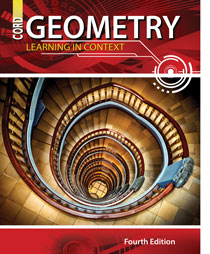Welcome to CORD Online Resources For Learning in Context

Learning in Context is an on-line supplement for our math and science textbooks. Each lesson in CORD’s textbooks is supported by a series of links to various websites. These links add new or more in-depth meaning to the lessons taught in the textbook.

You can navigate each book by using the expanding menu at the left or clicking on the chapters below.

Chapter Index1.1 Basic Geometric Figures

Chapter 1: Discovering Geometry

Chapter 2: Reasoning and Proof

Chapter 3: Coordinate Geometry

Chapter 4: Transformational Geometry

Chapter 5: Triangle Theorems

Chapter 6: Similar Triangles

Chapter 7: Right Triangles